# Monomial

(diff) ← Older revision | Latest revision (diff) | Newer revision → (diff)

The simplest form of an algebraic expression, a polynomial containing only one term.

Like polynomials (see Ring of polynomials), monomials can be considered not only over a field but also over a ring. A monomial over a commutative ringin a set of variables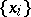, whereruns through some index set, is a pair, whereandis a mapping of the setinto the set of non-negative integers, wherefor all but a finite number of. A monomial is usually written in the form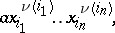where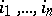are all the indices for which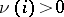. The number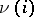is called the degree of the monomial in the variable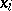, and the sum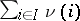is called the total degree of the monomial. The elements of the ring can be regarded as monomials of degree 0. A monomial withis called primitive. Any monomial with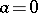is identified with the element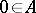.

The set of monomials overin the variables,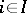, forms a commutative semi-group with identity. Here the product of two monomialsand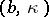is defined as.

Letbe a commutative-algebra. Then the monomial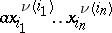defines a mapping of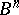intoby the formula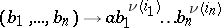.

Monomials in non-commuting variables are sometimes considered. Such monomials are defined as expressions of the formwhere the sequence of (not necessarily distinct) indicesis fixed.